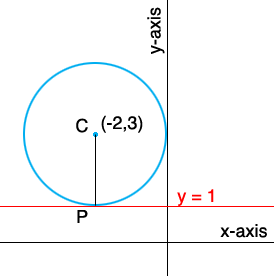SEARCH HOMEMath Central Quandaries & QueriesQuestion from crystal, a student: find the standard form of the circle with center (-2,3) and tangent to the line y=1Hi Crystal,The point P is the point on the circle where the tangent lie is y = 1. The line segment CP is perpendicular to the line y = 1. Do you know why?

What is the distance from P to C? This is the radius of the circle.

Can you write the equation of the circle with this radius and centered at (-2, 3)?

PennyMath Central is supported by the University of Regina and The Pacific Institute for the Mathematical Sciences.